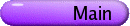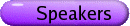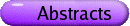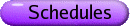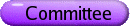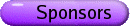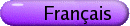Variational Analysis and Optimization
Org: Jiming Peng (MacMaster) and Jane Ye (Victoria)
[PDF]

DIDIER AUSSEL, University of Perpignan, 52 av. P. Alduy, 66860 Perpignan Cedex, France
On quasiconvex mathematical programming problems with equilibrium constraints
[PDF]

A mathematical programming problem with equilibrium constraints (MPEC) is a constrained optimization problem with equality and inequality constraints and, additionally, some equilibrium constraints:

 (MPEC)
 min f(z)
s.t.
 g(z) £ 0,
 h(z)=0,
 G(z) ³ 0, H(z) ³ 0,
 áG(z), H(z) ñ = 0
In general the constraint region associated to this problem is neither compact nor convex, even if the constraint functions are supposed to be linear. Nevertheless under the weak assumption that g is quasiconvex and h, H and G are quasiaffine, the constraint set appears to be locally starshaped.

The locally starshapedness is a rich structure and we will first present some existence results, necessary and sufficient optimality conditions for the problem of minimizing a quasiconvex function over a locally starshaped set.

Corresponding results for quasiconvex MPECs will be obtained as particular cases.

## References


D. Aussel and J. Ye, Quasiconvex programming with locally starshaped constraint region and applications to quasiconvex MPEC. Optimization, to appear.


D. Aussel and N. Hadjisavvas, Adjusted sublevel sets, normal operator and quasiconvex programming. SIAM J. Optim., to appear.

HEINZ BAUSCHKE, UBC Okanagan
Fitzpatrick functions and (cyclically) monotone operators
[PDF]

In 1988, Simon Fitzpatrick defined a new convex function FA-nowadays called the Fitzpatrick function-associated with a monotone operator A, and similarly a monotone operator Gf associated with a convex function f.

This talk surveys recent joint works with Alex McLaren (Guelph) and Hristo Sendov (Guelph), and with Jon Borwein (Dalhousie) and Shawn Wang (UBC Okanagan).

We consider the Fitzpatrick function of the subdifferential operator of a proper, lower semicontinuous, and convex function. This operator is cyclically monotone. A refinement of the classical Fenchel-Young inequality is derived and conditions for equality are investigated. The results are illustrated by several examples.

We also study the problem, originally posed by Fitzpatrick, of determining when A=GFA. Fitzpatrick proved that this identity is satisfied whenever A is a maximal monotone; however, he also observed that it can hold even in the absence of maximal monotonicity. We propose a new condition sufficient for this identity, formulated in terms of the polarity notions introduced recently by Martinez-Legaz and Svaiter.

JIM BURKE, University of Washington
Variational Analysis and the Roots of Polynomials
[PDF]

In a number of applications to stability and control one is interested in the behavior of certain functions of the roots of a polynomial as the polynomial varies. For example, how does the maximum modulus of the roots of a polynomial change as the coefficients of the polynomial varies?

In this talk we show how convex the geometry of the sets of roots of a polynomial and its derivative can be used to give insight into the variational behavior of such functions.

RICK CARON, University of Windsor, Windsor, ON
Random methods for the analysis of quadratic constraint sets
[PDF]

We review a framework for the analysis of sets of constraints, with no explicit assumptions, and we show the connection between minimal representations, irreducible infeasible systems, minimal infeasibility sets, as well as other attributes of the preprocessing of mathematical programs.

We show how the framework facilitates the development of a probabilistic preprocessing algorithm for a variety of mathematical programs, and we apply it specifically to convex quadratic constraint sets. We show, by example, that the method is surprisingly effective in finding feasible solutions.

Co-author: Dr. Tim Traynor, University of Windsor.

JOHN DENNIS, Rice University and University of Washington
[PDF]

The Mesh Adaptive Direct Search (MADS) class of derivative-free algorithms is effective for industrial strength nonlinear inequality constrained optimization. It has been incorporated into the MATLAB GADS package.

MADS is the direct result of applying nonsmooth analysis to GPS, an earlier class of similar algorithms. This powerful tools of nonsmooth analysis made clear GPS deficiencies glossed over by assuming smoothness. MADS has a satisfying convergence theory based on the Clarke calculus and Rockafeller's notion of a hypertangent cone.

MADS replaced GPS in our NOMAD software presently in use by our industrial collaborators. It is applicable to a wider class of problems than GPS, including yes/no constraints, and in all trials to date, MADS is more reliable and efficient.

This research was done in collaboration with Professor Charles Audet of Ecole Polytechnique de Montréal.

YUYING LI, University of Waterloo, 200 University Avenue West, Waterloo, ON
A Graduated Non-convexity Method for Applications in Finance
[PDF]

Investment and risk management in finance often require determining a small number of assets to minimize some measure of tracking error. Determining such a solution requires solving a mixed non-linear integer programming problem. We propose a graduated non-convexity method to minimize portfolio tracking error with the total number of assets no greater than a specified integer K. The solution of this tracking error minimization problem is the global minimizer of the sum of the tracking error function and the discontinuous counting function. We attempt to track the globally minimal tracking error portfolio by approximating the discontinuous counting function with a sequence of continuously differentiable non-convex functions, a graduated non-convexity process. We discuss the advantages of this approach and present numerical results in index tracking and hedging under basis risk applications in finance.

YVES LUCET, University of British Columbia Okanagan, 3333 University Way, Kelowna, BC V1V 1V7
Fast Moreau Envelope Algorithms and Applications
[PDF]

We present a new algorithm (named NEP for NonExpansive Proximal mapping) to compute the discrete Moreau envelope Ml,X (s) = minx Î X [[(|| s-x ||2)/(2l)] + f(x)] of a function f, where X is a discrete grid and l > 0. Numerical comparisons between the NEP and two existing algorithms: The Linear-time Legendre Transform (LLT) and the Parabolic Envelope (PE) algorithms will be shown along with worst-case time complexity, convergence results, numerical comparison, and examples.

The algorithms will be applied to compute numerical solutions to Hamilton-Jacobi equations, and the distance transform of image processing.

## References


Y. Lucet, Faster than the Fast Legendre Transform, the Linear-time Legendre Transform. Numer. Algorithms 16(1997), 171-185.


, Fast Moreau Envelope computation I: Numerical algorithms. Technical Report, University of British Columbia Okanagan, 2005.


, A linear euclidean distance transform algorithm based on the Linear-time Legendre Transform. In: Proceedings of the Second Canadian Conference on Computer and Robot Vision (CRV 2005), Victoria, BC, May 2005, IEEE Computer Society Press.

JIMING PENG, McMaster University, Hamilton, Ontario
0-1 Semidefinite Programming for Clustering: Modeling and Approximation
[PDF]

In the past few years, spectral clustering has caught much attention in the machine learning community and numerous algorithms have been proposed and well-studied. In this paper, we present a unified framework for these methods based on a new optimization model: 0-1 semidefinite programming (0-1 SDP). We also show that various constrained clustering problems can be embedded into the new 0-1 SDP model. Secondly, we consider the issue of how to solve the underlying 0-1 SDP problem. We consider two approximation methods based on principal component analysis (PCA) and projected PCA, respectively and prove that both algorithms can provide a 2-approximation to the original clustering problem. The complexity of these approximation algorithms are also discussed.

MOHAMED TAWHID, Thompson Rivers University
On some applications of H-differentiability to optimization and complementarity problems
[PDF]

In this talk, we consider two applications of H-differentiability. In the first application, we derive a necessary optimality condition for a local minimum of an H-differentiable function. In the second application, we consider a non-linear complementarity problem corresponding to an H-differentiable function f and show how, under appropriate conditions on an H-differential of f, minimizing a merit function corresponding to f leads to a solution of the non-linear complementarity problem. These two applications were motivated by numerous studies carried out for C1, convex, locally Lipschitzian, and semismooth function by various researchers.

PAUL TSENG, University of Washington
SOCP Relaxation of Sensor Network Localization
[PDF]

We study a second-order cone programming (SOCP) relaxation of the NP-hard sensor network localization problem. We show that SOCP relaxation, though weaker than SDP relaxation, has nice properties that make it useful as a problem preprocessor. In particular, an error bound result shows that sensors that are uniquely positioned among interior solutions of the SOCP relaxation are accurate up to the square root of the distance error. Thus, these sensors, which can be easily identified, are accurately positioned. In our numerical simulation, the interior solution found can accurately position up to 80-90% of the sensors. We also propose a smoothing coordinate gradient descent method for finding an interior solution faster than using SeDuMi.

XIANFU WANG, University of British Columbia: Okanagan
The extremal characterization of reflexive spaces
[PDF]

Assume that a Banach space has a Fréchet differentiable and locally uniformly convex norm. We show that the reflexive property of the Banach space is not only sufficient but also a necessary condition for the fulfillment of the proximal extremal principle in non-smooth analysis.

HERRE WIERSMA, Dalhousie University
Asplund Decomposition of Monotone Operators
[PDF]

A somewhat overlooked 1970 paper by Edgar Asplund establishes a decomposition for a maximal monotone operator T : X®X* on a general Banach space X. The operator T is decomposed as the pointwise sum of the (well-behaved) subdifferential mapping f of a convex function f, and an `acyclic' component A. I will reproduce a modern version of this result, and summarize current knowledge of the properties of this acyclic part.

HENRY WOLKOWICZ, Faculty of Mathematics, University of Waterloo, Waterloo, Ontario
Approximate and Exact Completion Problems for Euclidean Distance Matrices using Semidefinite Programming
[PDF]

A partial pre-distance matrix A is a matrix with zero diagonal and with certain elements fixed to given nonnegative values; the other elements are considered free. The EDM completion problem chooses nonnegative values for the free elements in order to obtain a Euclidean distance matrix, EDM. The nearest (or approximate) EDM problem is to find a Euclidean distance matrix that is nearest in the Frobenius norm to the matrix A, when the free variables are discounted.

Applications for EDM include: molecular conformation problems in chemistry; multidimensional scaling and multivariate analysis problems in statistics; sensor localization; genetics, geography, etc.

We present two algorithms: one for the exact completion problem and one for the approximate completion problem. Both use a reformulation of EDM into a semidefinite programming problem, SDP. The first algorithm is based on an implicit equation for the completion that for many instances provides an explicit solution. The other algorithm is based on primal-dual interior-point methods that exploit the structure and sparsity. Included are results on maps that arise that keep the EDM and SDP cones invariant.

We conclude with numerical tests.

JANE YE, University of Victoria
Optimality conditions for generalized semi-infinite programming problems
[PDF]

In this talk we consider the first order optimality conditions for the class of generalized semi-infinite programming problems (GSIPs). We extend the well-known constraint qualifications for finite programming problems such as the calmness condition, the Abadie constraint qualification, the Kuhn-Tucker constraint qualification, the Zangwill constraint qualification, the Slater condition, the linear independence constraint qualification and the weak reverse convex constraint qualification to GSIPs and analyze the extent to which a corresponding Karush-Kuhn-Tucker condition depends on these extensions. Relationships among various constraint qualifications are also given.

XIAOMING YUAN, University of Victoria, Victoria, BC V8W 3P4
Some hybrid methods for structured monotone variational inequalities
[PDF]

This talk presents some hybrid methods for solving monotone variational inequalities with separate structures. In particular, some descent directions are derived from the well-known alternating directions method and its variants and thus some hybrid methods are presented. The optimal step sizes along the descent directions also improve the efficiency of the new methods. Some numerical results demonstrate that the new methods are effective in practice.